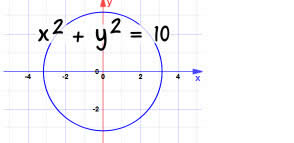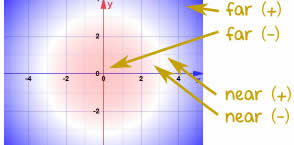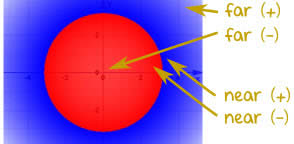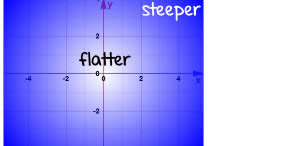# Equation Grapher (Flash Version)

Enter an Equation using the variables x and/or y and an =, press Go:

## Description

It can plot an equation where x and y are related somehow (not just y=...), like these:

### Examples:

• x^2+y^2=9 (an equation of a circle with a radius of 3)
• sin(x)+cos(y)=0.5
• 2x-3y=1
• cos(x^2)=y
• (x-3)(x+3)=y^2
• y=x^2

If you don't include an equals sign, it will assume you mean "=0"

It has not been well tested, so have fun with it, but don't trust it.

If it gives you problems, let me know.

Note: it can take a few seconds to finish, because it has to do lots of calculations.

If you just want to graph a function in "y=..." style you may prefer Function Grapher and Calculator

## Zooming

To zoom into an area, select an area with the mouse (click, hold down and drag, then release). The area you selected will be the new bounds.

If you just click-and-release (without creating a rectangle), then the spot you clicked on will be the new center

You can zoom out using the Out 2X or Out 10x button.

To reset the zoom to the original bounds click on the Reset button.

## Plot Styles

As well as the usual curve produced by Fast or Detailed:You can also have shaded plots of how far the value of the left hand side of the equation is from the right hand side:Or how near they are (very handy for finding where the equation may be true but the grapher just misses):And there is also an option for the slope of the equation:

This option takes a little longer to run (lots of calcs to do).

When the slope is large it shows as green.The usual thing applies: these are all plotted using computer calculations. Round-off can cause errors or values can be missed completely.

## All Functions

### Operators

+ Addition operator Subtraction operator Multiplication operator Division operator Exponent (Power) operator

### Functions

sqrt Square Root of a value or expression. sine of a value or expression cosine of a value or expression tangent of a value or expression inverse sine (arcsine) of a value or expression inverse cosine (arccos) of a value or expression inverse tangent (arctangent) of a value or expression Hyperbolic sine (sinh) of a value or expression Hyperbolic cosine (cosh) of a value or expression Hyperbolic tangent (tanh) of a value or expression e (the Euler Constant) raised to the power of a value or expression The natural logarithm of a value or expression The base-10 logarithm of a value or expression Returns the largest (closest to positive infinity) value that is not greater than the argument and is equal to a mathematical integer. Returns the smallest (closest to negative infinity) value that is not less than the argument and is equal to a mathematical integer. Round to the nearest integer. Examples: round(-2.5) = -2, round(-0.1) = 0, round(0.1) = 0, round(2.5) = 3 Absolute value (distance from zero) of a value or expression Sign (+1 or -1) of a value or expression

### Constants

pi The constant π (3.141592654...) Euler's number (2.71828...), the base for the natural logarithm